Next: Parametric Mappings Up: General Assumptions Previous: General Assumptions

#### Retinocortical Transformation

In functional terms, retinocortical pathway can be modeled as a computational channel from a source-layer and a target-layer. The computation is specified as a transformation mapping that relates stimulations on the source-layer to patterns of excitation on the target-layer. These layers represent respectively the visual field (S) and the cortical plane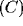, where simple cells are supposed uniformly distributed. Assumed the homogeneity and continuity of the layers and the linearity of simple cell responses, we can describe the behavior of the system by using linear operator theory. Thus, if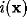is the visual input, the spatial distribution of excitation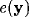on the cortical plane can be written as: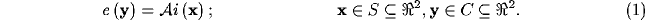where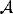is an integral linear operator of kernel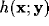: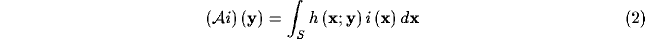Next: Parametric Mappings Up: General Assumptions Previous: General Assumptions